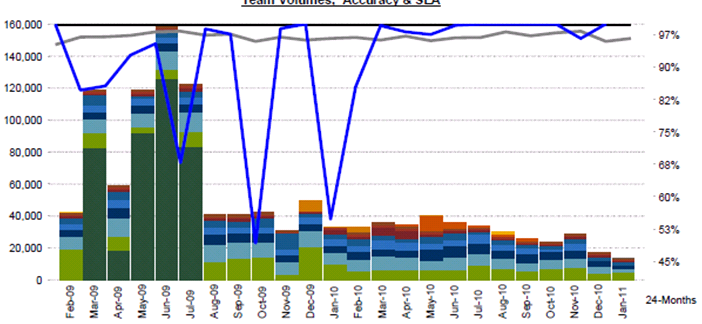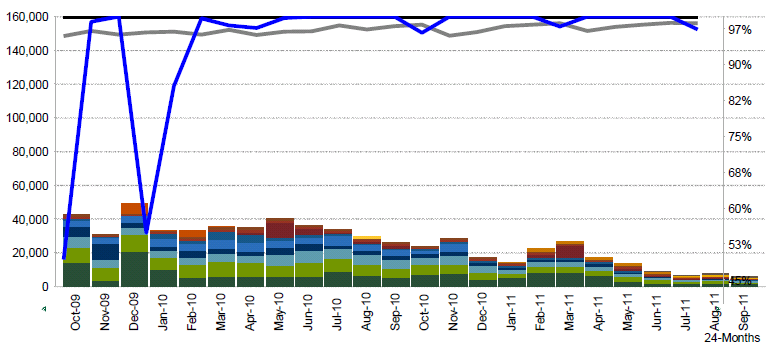# New to QlikView

Discussion board where members can get started with QlikView.Not applicable

## Graph Problem

Hello,

Please help, I was wondering how to fix the graph below.

Here's my graph last January 2011and here's my graph for this month. The scale is too high.Here's the expressions I used

Static Max:

=ceil(max(aggr(sum([Volumes]),[MMM-YY])),   pow(10,len(textbetween(round(max(aggr(sum([Volumes]),[MMM-YY]))),'','',1))-2))

Static Step:

=ceil(max(aggr(sum([Volumes]),[MMM-YY])),   pow(10,len(textbetween(round(max(aggr(sum([Volumes]),[MMM-YY]))),'','',1))-2))/8

Thanks,

Salie

5 RepliesMVP

## Graph Problem

Salie

Have you checked to see what these subexpressions evaluate to?

max(aggr(sum([Volumes]),[MMM-YY]))

and

pow(10,len(textbetween(round(max(aggr(sum([Volumes]),[MMM-YY]))),'','',1))-2))

If you need to see what values are returned by the aggregation:

concat(aggr(sum([Volumes]),[MMM-YY]), ',')

Regards

Jonathan

Logic will get you from a to b. Imagination will take you everywhere. - A EinsteinNot applicable

## Graph Problem

Hi Jonathan,

Where should I add

concat(aggr(sum([Volumes]),[MMM-YY]), ',')?

Thanks,

SalieNot applicable

## Re: Graph Problem

Hi Jonathan,

Where should I add

concat(aggr(sum([Volumes]),[MMM-YY]), ',')?

Thanks,

SalieMVP

## Graph Problem

Salie

Create a text box, and enter the expression in the text box. Put an = in front of the expression.

Regards

Jonathan

Logic will get you from a to b. Imagination will take you everywhere. - A Einstein
HighlightedNot applicable

## Re: Graph Problem

Hi,

I changed my expression to your expression. It worked. The only problem I have is that I need to show '50,000' after '45,000' to make it look complete.

I place the =concat expression you have provided on both Static Step and Static Max.thanks a lot!